Courses

# Facts That Matter- Triangles Class 9 Notes | EduRev

## Mathematics (Maths) Class 9

Created by: Full Circle

## Class 9 : Facts That Matter- Triangles Class 9 Notes | EduRev

The document Facts That Matter- Triangles Class 9 Notes | EduRev is a part of the Class 9 Course Mathematics (Maths) Class 9.
All you need of Class 9 at this link: Class 9

Facts That Matter

• Two congruent figures have exactly the same shape and size.
• If two triangles are congruent, then their corresponding parts are equal.
• If two sides and included angle of one triangle are equal to two sides and the included angle of the other triangle, then the two triangles are congruent. [SAS congruence rule]
• If two angles and the included side of one triangle are equal to two angles and included side of the other triangle, then the two triangles are congruent. [ASA congruence rule]
• If two angles and one side of a triangle are equal to two angles and the corresponding side of the other triangles, then the two triangles are congruent. [AAS congruence rule]
• If three sides of one triangle are equal to three sides of other triangle, then the two triangles are congruent. [SSS congruence rule]
• If in two right triangles, hypotenuse and one side of a triangle are equal to the hypotenuse and one side of the other triangle, then the two triangles are congruent. [RHS congruence rule]
• Angles opposite to equal sides of a triangle are equal.
• Sides opposite to equal angles are equal.
• The measure of each angle of an equilateral triangle is 60º.
• In a triangle, the sum of any two sides is always greater than the third side.
• In a triangle, the angle opposite to the longer side is (greater) larger.
• In a triangle, the side opposite to the greater angle is longer.

CONGRUENT FIGURES
If two geometrical figures have exactly the same shape and size then they are called congruent figures.
Note: (i) Two line segments are congruent only when their lengths are equal.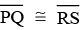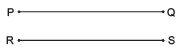(ii) Two angles are congruent only when their degree measures are equal. ∠ABC ≌ ∠PQR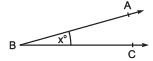(iii) The symbol ‘≌’ is used to represent congruence.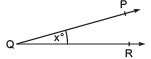CONGRUENT TRIANGLES
Two triangles are congruent if and only if one of them can be made to superimpose on the other, such as to cover it exactly.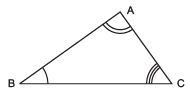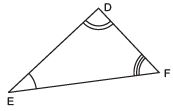In the figure, ΔABC ≌ ΔDEF.

Note: (i) When ΔABC ≌ ΔDEF, then sides of ΔDEF fall on corresponding equal sides of ΔABC, i.e.
DE covers AB or DE ↔ AB; EF covers BC or EF ↔ BC and FD covers CA or FD ↔ CA.
(ii) In case ΔABC ≌ ΔDEF,
∠D covers ∠A or ∠D ↔ ∠A.
∠E covers ∠B or ∠E ↔ ∠B.
∠F covers ∠C or ∠F ↔ ∠C.
(iii) In case ΔABC ≌ ΔDEF
D corresponds to A or D ↔ A.
E corresponds to B or E ↔ B.
F corresponds to C or F ↔ C.
(iv) In congruent triangles, corresponding parts are equal and we write in short ‘c.p.c.t.’ “for Corresponding Parts of Congruent Triangles”.

CRITERIA FOR CONGRUENCE OF TRIANGLES
1. SAS Criteria:
If two triangles are such that two sides and the included angle of the one equal to the corresponding sides and the included angle of the other, then the triangles are congruent.
In the figure, we have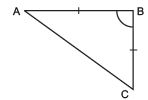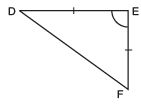AB = DE, BC = DF and ∠B = ∠E
ΔABC ≌ ΔDEF.
Note: It is necessary to write the correspondence of vertices correctly for writing of congruence of triangles in symbolic form.
In case of ΔABC ≌ ΔDEF, we have
A ↔ D, B ↔ E and C ↔ F.

II . ASA Criteria:
If two triangles are such that two angles and the included side of one are equal to the corresponding two angles and the included side of the other, then the two triangles are congruent. In the figure, we have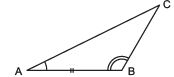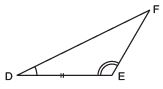∠A = ∠D; ∠B = ∠E and AB = DE
ΔABC ≌ ΔDEF.

Note: In case, in two triangles, two pairs of angles and one pair of corresponding sides are equal (even if the side is not included between the corresponding equal pairs of angles), the triangles are congruent.
This is because the sum of three angles of a triangle is 180°, therefore, if two pairs of angles are equal, the third pair is also equal (180° - sum of equal angles). Thus, two triangles are congruent if any two pairs of angles and one pair of corresponding sides are equal.
We may call it as the AAS congruence rule.

,

,

,

,

,

,

,

,

,

,

,

,

,

,

,

,

,

,

,

,

,

;